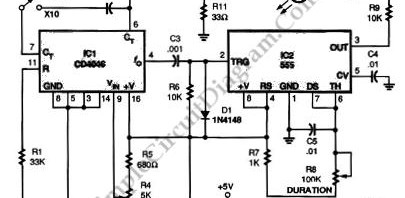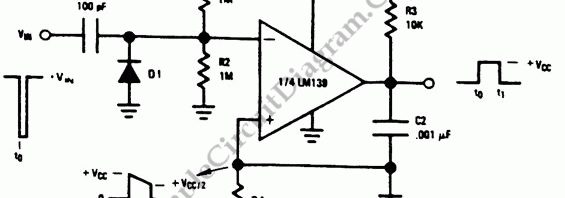## Simple Ultrasonic Wave GeneratorUltrasonic sound wave can be generated using electronic circuit. This simple electronic circuit can generate an ultrasonic wave with frequency range of 12 to 50 kHz. Its very easy and quick to make this circuit. This circuit uses NE555 IC timer to drive a speaker. SPKR1 can be piezzo tweeter, etc. If you use a moving coil tweeter, then it […]

## Solid-State (555 IC) StroboscopeStroboscope is used to measure the speed of rotation, using the effect of strobed light, where a moving object will be perceived as stationary object if the light is strobed fast enough relative to the movement speed. When applied to a rotating object, a mark on the object will be perceived as not rotating if the frequency of the strobed […]

## Voltage to Frequency Converter Using UJTThe following voltage to frequency converter (V/FC – VCO) circuit consist of a UJT (uni-junction transistor) oscillator in which the timing charge capacitor C2 is linearly depends on the input signal voltage. The voltage across resistor R5 set the charging current, which is accurately controlled by the amplifier. Here is the schematic diagram of the circuit: